Disclosure: This post may contain affiliate links, meaning when you click the links and make a purchase, we receive a commission.

# Separate, Split & Remove Substring & Number from Text with Excel Functions & VBA

[Editor’s Note: This is a guide on how to separate, split & remove substring & numbers from text using Excel Functions and VBA. Examples of substring functions are CHAR, FIND, LEFT, LOWER,  MID, PROPER, REPLACE, RIGHT, SEARCH, TRIM and UPPER. This article is written by Zhiping Yan, ExcelDemy’s regular contributor. Any feedback is welcome. If you find any mistake or grammatical error, let us know in the comments section. Your valuable feedback will help us to update this guide with time to time.]

Excel provides many functions that can be used to manipulate strings. These functions are very useful especially when you have to clean data. This article will show you those functions and relevant examples.

## Change the case of text

With UPPER, LOWER or PROPER function, you can change the case of text to uppercase, lowercase, or proper case. UPPER function can change a text to all uppercase. LOWER function can change a text to all lowercase while the PROPER function will capitalize the first letter in each word. These functions are very simple and Figure 1.1 shows how to use them.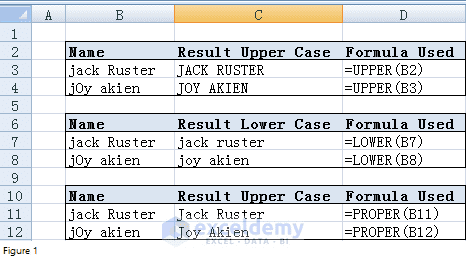Figure 1

## Yield special characters

CHAR function can convert a number (from 1 to 255) into ASCII character. For example, CHAR(65) yields A and CHAR(66) returns B. Figure 2.1 and Figure 2.2 shows you the code number and corresponding character. You can see that CHAR function is useful when you want to type characters that are awkward or impossible to type directly. Please note that Excel will return “#VALUE!” error (cell N35) if the code number is greater than 255. Now let’s look at the cells highlighted in yellow; Excel CHAR function returns nothing. What happened? CHAR(9) represents a horizontal tab and horizontal tab is invisible. That is the reason why the cell is blank.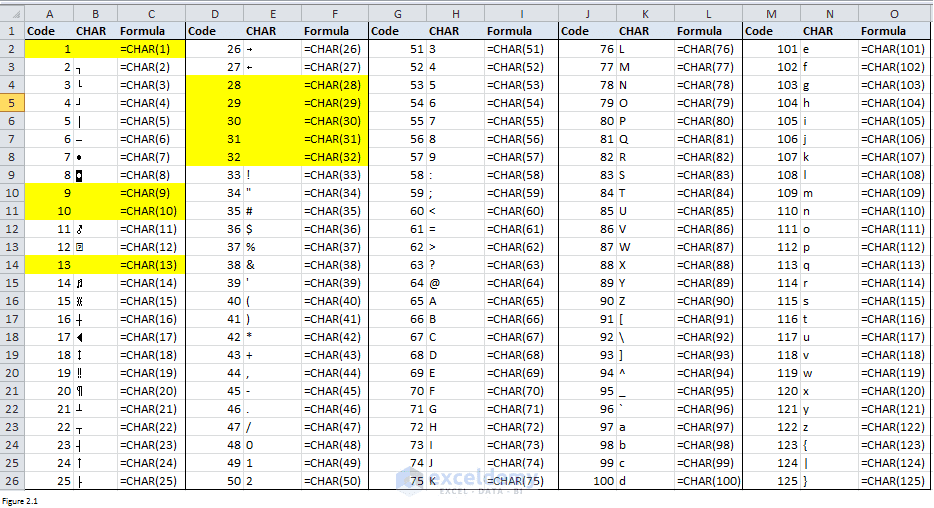Figure 2.1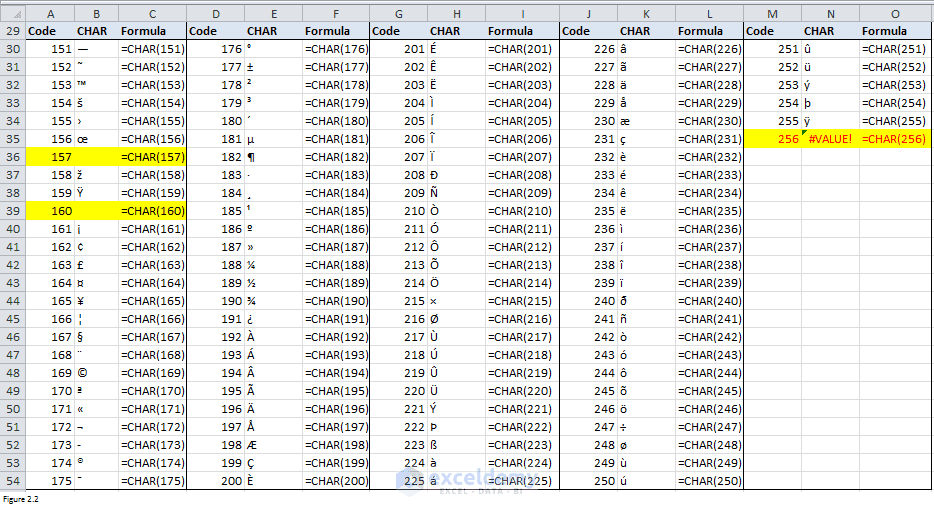Figure 2.2

If we use REPLACE function to replace “,” with CHAR(10), you will see that “take me home” was shifted to another line (Figure 2.3). It tells that CHAR function can convert 10 into a new line. Observe range B7:C8; you see that both CHAR(32) and CHAR(160) return blank spaces. CHAR(1) also returns a blank space (range B4:C4). CHAR(13) returns a carriage return.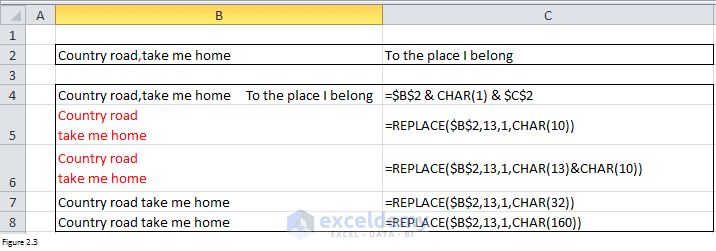Figure 2.3

## Remove blank spaces

TRIM function can be used to remove all spaces from a text string except for single spaces between words. It is well known that TRIM function can remove leading and trailing spaces. Moreover, it can also replace multiple consecutive spaces with single space. For example, there is only one blank space between “stranger” and “to” in cell C4 after applying the TRIM function to cell B4.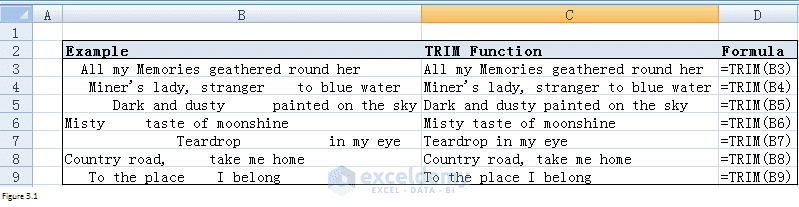Figure 3.1

## Return the number of characters in a string

LEN function can be used to return the number of characters in a text string. This function is simple. For example, LEN(“abc 123”) will return 7. Here I have to remind you that blank spaces will also be counted.

## Extract text from another text string

### LEFT/MID/RIGHT

Functions such as LEFT, RIGHT, MID can help you extract a word or text from another text string. Following table shows you the syntax of those functions.

 LEFT LEFT(text, [num_chars])Returns first num_chars characters in text string. RIGHT RIGHT(text, [num_chars])Returns last num_chars characters in text string. MID MID( string, start [, length ])Returns a string containing a specified number of characters from the start position of a string.

We have two strings in column B. What we need to do is to extract and put name, height, weight in column C, E and G respectively. Figure 4.1 shows you how to use LEFT, MID, and RIGHT function to fulfill this task. But if you look at the formulas closely, you will find that we need to change arguments each time the position changes. For example, the second argument should be 10 if we need to extract “Name: Jack”. But the argument turns to be 11 when coming to “Name: Marie”. It is annoying, right?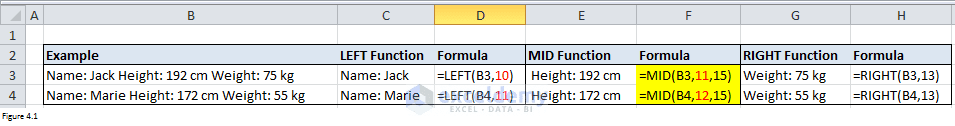Figure 4.1

### Combination of LEFT/MID/RIGHT with FIND/SEARCH

Luckily, we have another choice. The combination of these functions with FIND/SEARCH function can help you deal with complicated problems. Before showing the example, I will give you the syntax for FIND and SEARCH functions.

 FIND FIND(find_text, within_text, [start_num])Locates find_text string within within_text and returns the number of the starting position of the find_text from the first character of the second wthin_text. Start_num is optional. It is the number in the within_text argument at which you want to start searching. FIND function is case-sensitive. SEARCH SEARCH(find_text, within_text, [start_num])Locates find_text string within within_text and returns the number of the starting position of the find_text from the first character of the second wthin_text. Start_num is optional. It is the number in the within_text argument at which you want to start searching. The SEARCH function is not case-sensitive.

Formula “FIND(“Height”,\$B7,1)” returns the position of “Height” within the string in cell B7. It is 12 and it is the position of “H” (the first letter of “Height”) as a matter of fact. There is one blank space between “Name: Jack” and “H”. Therefore, “FIND(“Height”,\$B7,1) – 2” can is, in essence, the length of “Name: Jack”. Thus, “=LEFT(\$B7,FIND(“Height”,\$B7,1)-2)” can return the “Name: Jack”. Copy this formula into cell C8, we can extract the name for the second person. Figure 4.2 also shows you how to combine MID/RIGHT function with FIND function to retrieve text in middle part of the right part.

Figure 4.3 shows you that FIND function is case-sensitive while SEARCH function is not case-sensitive. You need to be careful when determining which function should be used.

### Split text and digits – 1

Suppose that we have scores for Jack, Marie and Hurry in column B. How can we split name and score? Formula “=MIN(FIND({0,1,2,3,4,5,6,7,8,9},B3&”0123456789″))” can return the position of the first digit within string “Jack94”. And with this returned position number, we can extract text and score using LEFT and RIGHT formula respectively. Here is where you can find the details about array formula.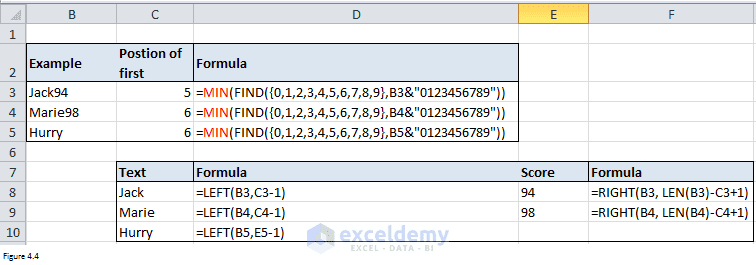Figure 4.4

Now let’s see what does “FIND({0,1,2,3,4,5,6,7,8,9},B3&”0123456789″)” will return. Put “Jack940123456789” which is the result of the concatenation of “Jack94” and “0123456789” in cell F1. Select range G3:P3 and enter “=FIND({0,1,2,3,4,5,6,7,8,9},B3&”0123456789″)” in cell G3 and then enter CTRL+SHIFT+ENTER, excel will return numbers in red box as below in Figure 4.5. As a matter of fact, range G3:P3 returns the position of “0”, “1”…”9” in string “Jack940123456789”. If you enter “FIND(G1,F1)” in cell G2, you will get the same number as that in cell G3. By copying this formula into range H2:P2, you will find that numbers in range “H2:P2” are the same as those in range “H3:P3”.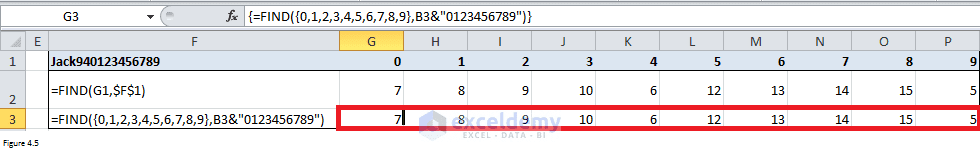Figure 4.5

You can see that the position of first “9” in string “Jack940123456789” is 5. And 5 is the smallest number in range G3:P3. That’s the reason why MIN function is applied here.

### Split text and digits – 2

Sometimes, letters and digits are placed together in a way that you cannot find a rule to split them easily. In this case, we can use VBA code to complete this task. Let’s take Figure 4.6 as an example. By clicking on Split button in Figure 4.6, Excel can extract letters from column A and put them in column B. Digits will be entered into column C while other special characters will be put in column D.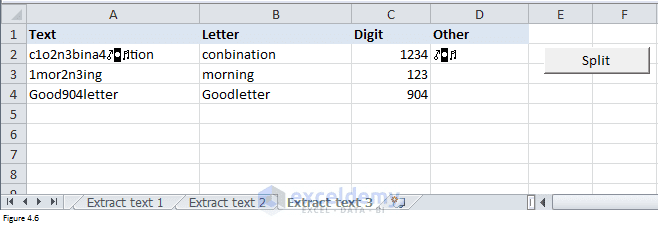Figure 4.6

Here is the code. Generally, MID function will be used to pick out each character first. If the character is a letter, it will be put at the end of String A and it will be put at the end of String B if the character is a digit. Otherwise, it will be placed at the end of String C. Finally, Excel will put String A, String B and String C in column B, C, and D respectively.

 Source code```Sub extract_click()

Dim StrA As String

Dim StrB As String

Dim StrC As String

Dim str As String

ThisWorkbook.Worksheets("Extract text 3").Activate

nrow = ActiveSheet.Range("A65536").End(xlUp).Row

'clean previous data

For i = 2 To nrow

For j = 2 To 4

ActiveSheet.Cells(i, j) = ""

Next j

Next i

For i = 2 To nrow

str = ActiveSheet.Cells(i, 1)

For j = 1 To Len(str)

temp = Mid(str, j, 1)

If temp Like "[A-Za-z]" Then

StrA = StrA & temp

ElseIf temp Like "[0-9]" Then

StrB = StrB & temp

Else

StrC = StrC & temp

End If

Next j

ActiveSheet.Cells(i, 2) = StrA

ActiveSheet.Cells(i, 3) = StrB

ActiveSheet.Cells(i, 4) = StrC

StrA = ""

StrB = ""

StrC = ""

Next i

End Sub```

“ThisWorkbook.Worksheets(“Extract text 3”).Range(“A65536”).End(xlUp).Row” can return the last used row number. By applying this number to for loop, Excel can split as many as strings as long as you put them in column A.

Below code can clean previously extracted data from the second row in column B, C, and D. Thus what you need to do only is to update column A each time you want to split new strings.

 Source code```'clean previous data

For i = 2 To nrow

For j = 2 To 4

ActiveSheet.Cells(i, j) = ""

Next j

Next i```

### Split text and digits – 3

More than often, we do want the characters are concatenated like above when extracting. How can we extract text and digits in a way like below in Figure 4.7?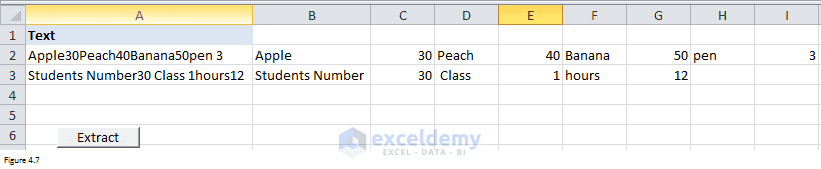Figure 4.7

Here is the VBA code which can enable Excel to complete this kind of task.

 Source code```Sub extract1_click()

Dim StrA As String

Dim str As String

ThisWorkbook.Worksheets("Extract text 4").Activate

nrow = ActiveSheet.Range("A65536").End(xlUp).Row

ncol = ActiveSheet.UsedRange.Columns.Count

'clean data

For i = 2 To nrow

For j = 2 To ncol

ActiveSheet.Cells(i, j) = ""

Next j

Next i

a = 2

For i = 2 To nrow

str = ActiveSheet.Cells(i, 1)

For j = 1 To Len(str)

temp1 = Mid(str, j, 1)

If j < Len(str) Then

temp2 = Mid(str, j + 1, 1)

Else

temp2 = Mid(str, j, 1)

End If

StrA = StrA & temp1

If ((Not (temp1 Like "[0-9]")) And temp2 Like "[0-9]") Or ((Not (temp2 Like "[0-9]")) And temp1 Like "[0-9]") Then

ActiveSheet.Cells(i, a) = StrA

StrA = ""

a = a + 1

End If

If j = Len(str) Then

ActiveSheet.Cells(i, a) = StrA

StrA = ""

End If

Next j

a = 2

Next i

End Sub```

The key point here is that it will pick up two characters at the same time. If the first character is not a digit and the second the character is a digit, Excel will stop concatenating and put the concatenated strings in the worksheet. Similarly, Excel will do the same thing if the first character is a digit and the second the character is not a digit. If Excel reaches the last character in the string, it will put the concatenated string into worksheet too. Please note that StrA will be set to be null once Excel stops concatenating or reaches the last character. In order to put extracted text or number into different cells, a will be added by 1 each time Excel stops concatenating. It will be set to be 2 once Excel reaches the last character since Excel will move to a new row and start splitting new strings.

### Split text separated by fixed symbol

Sometimes the substrings may be separated by a fixed symbol like “~”. By clicking on Split button, we can extract substrings and put each of them in different cells.

Here is the VBA code. The key point here is the SPLIT function. It is simple and I will not discuss in details.

 Source code```Sub extract2_click()

ThisWorkbook.Worksheets("Extract text 5").Activate

nrow = ActiveSheet.Range("A65536").End(xlUp).Row

ncol = ActiveSheet.UsedRange.Columns.Count

'clean data

For i = 2 To nrow

For j = 2 To ncol

ActiveSheet.Cells(i, j) = ""

Next j

Next i

'split

If ActiveSheet.Cells(1, 3) > "" Then

For i = 2 To nrow

For j = 0 To UBound(Split(ActiveSheet.Cells(i, 1), ActiveSheet.Cells(1, 3)))

temp = Split(ActiveSheet.Cells(i, 1), ActiveSheet.Cells(1, 3))(j)

ActiveSheet.Cells(i, 2 + j) = temp

Next j

Next i

Else

For i = 2 To nrow

For j = 0 To UBound(Split(ActiveSheet.Cells(i, 1), " "))

temp = Split(ActiveSheet.Cells(i, 1), " ")(j)

ActiveSheet.Cells(i, 2 + j) = temp

Next j

Next i

End If

End Sub```

Please note that the separated character can be changed. For example, if you change it to “S”, you will get different results as shown in Figure 4.9.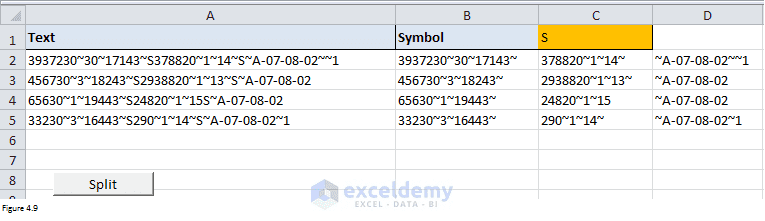Figure 4.9

Here is another example. After splitting, you can use SUM function to get the total of numbers within a specific string. For example, by using formula “=SUM(B3:D3)”, we can get 120.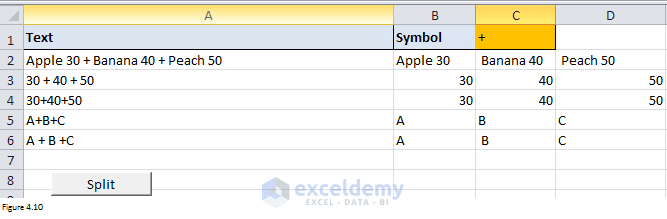Figure 4.10

Finally, here is the last example. If you set the cell C1 to be blank, Excel will consider the split symbol is a blank space. Please note that if you use macro in section Split text and digits – 3, you will get two strings “Students Number” and “30”. But using the macro in this section, you will get three strings: Students, Number and 30. Please select the appropriate macro for your own problem.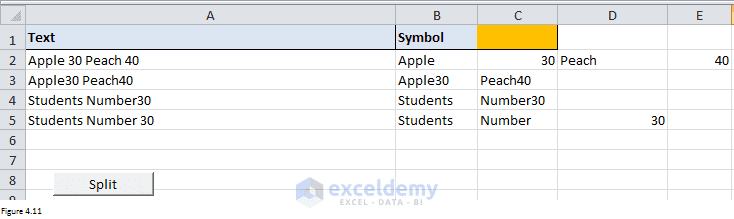Figure 4.11

## Concatenate text

Sometimes, we many want to join strings into one string. For the kind of problem, we can use CONCATENATE function. Here is the syntax:

 CONCATENATE CONCATENATE(text1, [text2], …)Join two or more text string into one string.

It is easy and I will make only one simple example. As a programmer, I have to deal with IF…Elseif often. What I usually do is to use CONCATENATE function to make whole statements (range D2:D6) and then copy them into Editor. You can use this function to solve your own problem.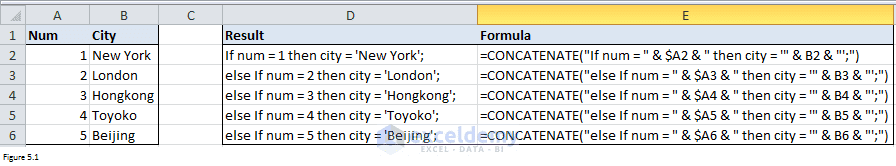Figure 5.1

I am from China and this photo was taken in a classical garden. There are many similar gardens in China, attracting a lot of visitors every year, especially in spring and summer.

I was major in Biotechnology. But I took a job as an SAS programmer because I prefer programming. Besides SAS, I also learned Excel VBA in my spare time. It is fantastic to be able to manipulate data, files and even to interact with the internet via programming. This will save me a lot of time. I am keen to learn new things.

1. Reply• Reply